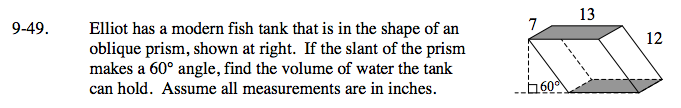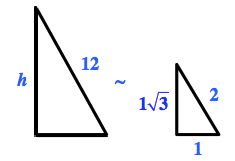### Home > GC > Chapter 9 > Lesson 9.1.5 > Problem9-49

9-49.Use a special right triangle to find the height of the parallelogram.
For help, see the Math Notes box in Lesson 5.3.1.

Find the height of the parallelogram:linear scale factor = 6

$h=6(1\sqrt{3})=6\sqrt{3}\approx 10.39 \text{ inches}$

Find the area of the base:

Area of parallelogram = area of prism base
Area of a parallelogram = (base)(height)
= (13)(7)
= 91 inches²

Find the volume of the prism:

Volume of prism = (area of base)(depth)
≈ (91 inches²)(10.39 inches)
≈ 945.49 inches³